# Protractor usagePage 1

#### WATCH ALL SLIDES

Slide 1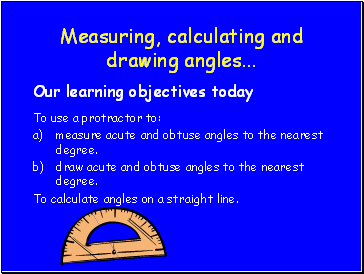## Measuring, calculating and drawing angles .

Our learning objectives today

To use a protractor to:

measure acute and obtuse angles to the nearest degree.

draw acute and obtuse angles to the nearest degree.

To calculate angles on a straight line.

Slide 2## What do we use to help us?

A protractor

Here is a standard protractor like you

use in the classroom.

Slide 3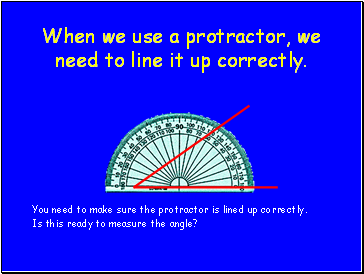## When we use a protractor, we need to line it up correctly.

You need to make sure the protractor is lined up correctly.

Is this ready to measure the angle?

Slide 4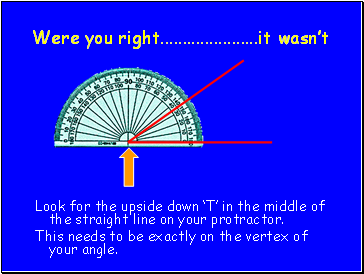Were you right it wasn’t

Look for the upside down ‘T’ in the middle of the straight line on your protractor.

This needs to be exactly on the vertex of your angle.

Slide 5We need to remember .

It doesn’t

matter which

way round the

angle is, you

ALWAYS need

to line the upside

down ‘T’ to the vertex

of the angle.

Slide 6Slide 7Once you reach 30° you need to be careful!!!

You then need to look at the 1° markings on the outer set of numbers.

Slide 8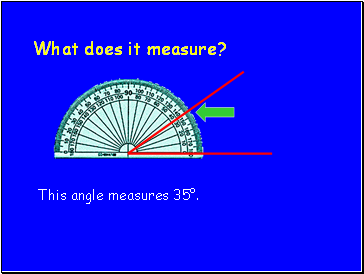## What does it measure?

This angle measures 35°.

Slide 9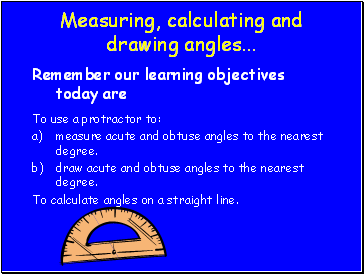## Measuring, calculating and drawing angles .

Remember our learning objectives today are

To use a protractor to:

measure acute and obtuse angles to the nearest degree.

draw acute and obtuse angles to the nearest degree.

To calculate angles on a straight line.